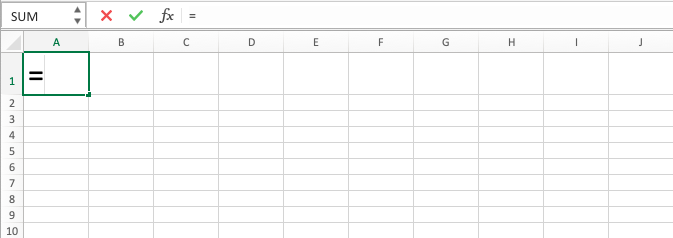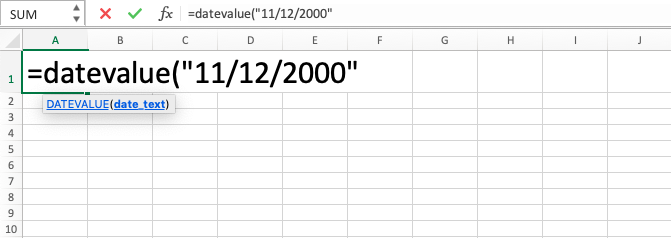DATEVALUE Function in Excel - Compute Expert

# DATEVALUE Function in Excel

Home >> Excel Tutorials from Compute Expert >> Excel Formulas List >> DATEVALUE Function in Excel

In this tutorial, you will learn what is and how to use DATEVALUE formula in excel. DATEVALUE formula in excel is useful to change a date in text form into its serial number representation in the spreadsheet. DATEVALUE function in excel is useful if we do data processing using a formula or pivot table that sometimes needs this kind of data as one of its processing factors.

## Why We Need to Learn About DATEVALUE Formula in Excel?

Date kind of data can be represented by the number of its days amount. It is the addition result of the days in its day, month, and year numbers that forms it. Sometimes if we have many data entries with one column consists of date kind of data in the spreadsheet, we need number representation based on the days’ amount from each of the column content to do our calculation or use it as one of the factors in our data processing. But, for sure, it will be troublesome if we need to do the number representation calculation for each of the data in the column manually because we need to separate day, month, and year digits from each of them first before the calculation can be correctly done.

One case example where this kind of number is needed in our processing is when we want to do large and small comparisons of dates in a spreadsheet. If we do it without changing each of them into pure digits, then it will be harder to do the comparison process. Besides, if we use pivot table feature in the spreadsheet and want to process the days’ digits fast from all of the data in the date column, then it will be easier if we change them first into their digit form based on their days’ amount and put that change process results into a particular column so the process can be simpler when we activate the pivot table feature.

Besides that, another case is maybe we want to do prioritization to many data entries by considering various factors where one of them is the entry’s date. In calculating the contribution of each factor to get the priority ranking, it can be better that we represent each factor with numbers so they can be multiplied with their weight before summed up with other factor numbers to get total numbers from which the large and small will be compared to see the factor priority rank. If the data form is still date, then it will be harder to do the total digit calculation for the prioritization based on all of the factors.

To help in the changing process to a pure digit form from a date, then you can use DATEVALUE function in excel. DATEVALUE formula in excel will help in changing the input of date data into pure digit form which represents the serial number of the date. Very useful if you often process data that has dates in it.

## What is DATEVALUE Function in Excel?

DATEVALUE function in excel is a function with the usage objective to change date data in a text form into its serial digit in the spreadsheet.

In DATEVALUE formula in excel, 1 January 1900 is a date with serial digit of 1, The serial digit of the dates after this will follow the days addition amount from 1 January 1900. If you give input before 1 January 1900, then DATEVALUE function in excel will result in #VALUE error.

Here is a little bit explanation about the input in this formula:

=DATEVALUE(date_text)

Note:
date_text = date in the form of text that you want to change to its excel serial number form

## How to Use DATEVALUE Formula in Excel?

The following will explain how to use DATEVALUE function in excel. DATEVALUE formula in excel is quite easy to use because it only needs one input in its writing. You must pay attention, though, to the input form in DATEVALUE function in excel which must be correct (date in text form) so it does not result in an error.

## Using DATEVALUE Function in Excel

1. Type equal sign ( = ) in the cell where you want to put the result in2. Type DATEVALUE (can be with large and small letters) and open bracket sign after =3. Type date between quote signs or cell coordinate where the date which you want to process is after open bracket sign. If you input this in the form of cell coordinate, make sure the cell content is in text form so this formula will not result in an error4. Type close bracket sign5. Press Enter
6. The process of DATEVALUE function in excel is done!## Exercise

After you have learned how to use the DATEVALUE formula in excel above, you can practice your understanding by doing the exercise below!

### Questions

The table in the exercise file is data to determine product sending priority from a company’s warehouse
1. Fill column no. 1 with the serial number representation of its parallel in column C! (All the date data in column C is still not in text form. Use TEXT formula to change it into text form! Look at the tutorial in the other part of Compute Expert blog if you still don’t understand how to use TEXT!)
2. Fill column no. 2 with the serial number representation of column C added up with the production quantity!
3. The sending priority is based on the ascending order on column 2. Which product is the number one priority? Mark the cell on column no. 3 which is parallel with the priority number one product using red color!

To change a date into text form for the input of DATEVALUE formula in excel, you can use TEXT formula to do it. You can learn the tutorial in another part of Compute Expert blog if you still don’t understand how to use it!

Get updated excel info from Compute Expert by registering your email. It's free!